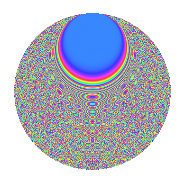# Properties

 Label 731.2.bhLevel 731 Weight 2 Character orbit bh Rep. character $$\chi_{731}(13,\cdot)$$ Character field $$\Q(\zeta_{84})$$ Dimension 1536 Newforms 1 Sturm bound 132 Trace bound 0

# Related objects

## Defining parameters

 Level: $$N$$ = $$731 = 17 \cdot 43$$ Weight: $$k$$ = $$2$$ Character orbit: $$[\chi]$$ = 731.bh (of order $$84$$ and degree $$24$$) Character conductor: $$\operatorname{cond}(\chi)$$ = $$731$$ Character field: $$\Q(\zeta_{84})$$ Newforms: $$1$$ Sturm bound: $$132$$ Trace bound: $$0$$

## Dimensions

The following table gives the dimensions of various subspaces of $$M_{2}(731, [\chi])$$.

Total New Old
Modular forms 1632 1632 0
Cusp forms 1536 1536 0
Eisenstein series 96 96 0

## Trace form

 $$1536q - 22q^{3} + 208q^{4} - 30q^{5} - 12q^{6} - 14q^{7} + O(q^{10})$$ $$1536q - 22q^{3} + 208q^{4} - 30q^{5} - 12q^{6} - 14q^{7} - 30q^{10} - 32q^{11} - 64q^{12} - 76q^{13} - 50q^{14} - 304q^{16} - 26q^{17} - 72q^{18} - 70q^{20} - 40q^{21} - 66q^{22} - 36q^{23} + 58q^{24} - 16q^{27} + 42q^{28} - 30q^{29} - 204q^{30} - 4q^{31} - 216q^{33} + 40q^{34} - 96q^{35} - 4q^{37} + 48q^{38} - 64q^{39} - 18q^{40} - 36q^{41} - 88q^{44} + 172q^{45} - 142q^{46} - 80q^{47} - 136q^{48} + 20q^{50} - 50q^{51} - 60q^{52} + 60q^{54} + 80q^{55} - 88q^{56} + 16q^{57} - 160q^{58} - 6q^{61} - 52q^{62} - 100q^{63} + 144q^{64} - 20q^{65} - 100q^{67} + 30q^{68} - 96q^{69} - 2q^{71} + 580q^{72} + 62q^{73} - 118q^{74} - 124q^{75} + 388q^{78} - 36q^{79} - 44q^{80} - 188q^{81} + 12q^{82} + 216q^{84} + 112q^{85} - 332q^{86} - 42q^{88} + 76q^{89} - 140q^{90} + 92q^{91} - 140q^{92} + 44q^{95} - 292q^{96} - 84q^{97} - 20q^{98} + 320q^{99} + O(q^{100})$$

## Decomposition of $$S_{2}^{\mathrm{new}}(731, [\chi])$$ into irreducible Hecke orbits

Label Dim. $$A$$ Field CM Traces $q$-expansion
$$a_2$$ $$a_3$$ $$a_5$$ $$a_7$$
731.2.bh.a $$1536$$ $$5.837$$ None $$0$$ $$-22$$ $$-30$$ $$-14$$